# TED_MSM.pptx

Mar. 22, 2023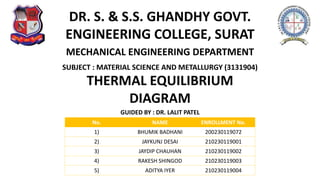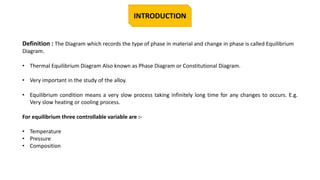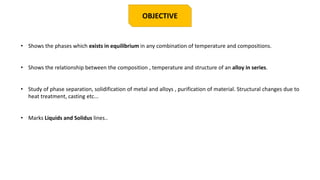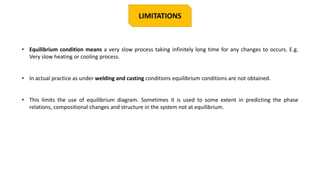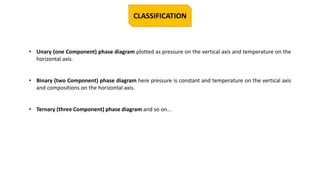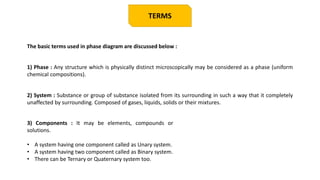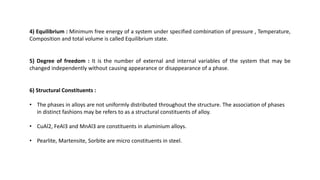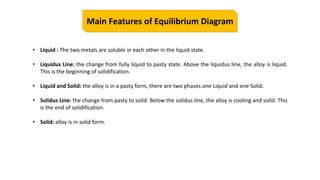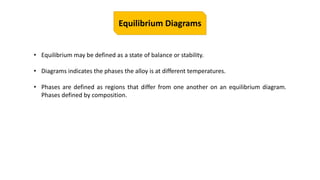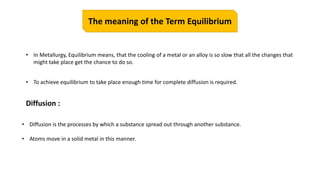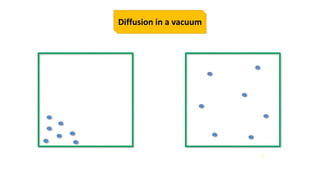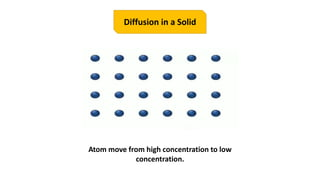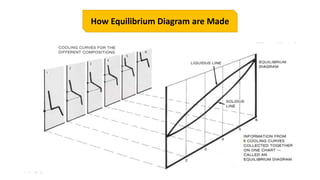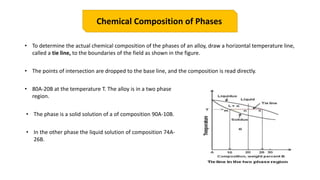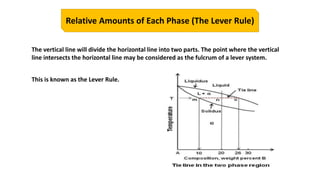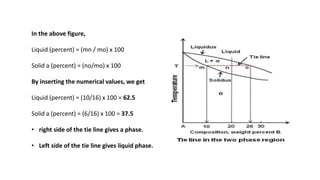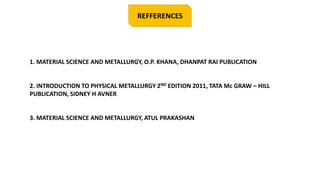1 of 18

### TED_MSM.pptx

1. DR. S. & S.S. GHANDHY GOVT. ENGINEERING COLLEGE, SURAT MECHANICAL ENGINEERING DEPARTMENT THERMAL EQUILIBRIUM DIAGRAM GUIDED BY : DR. LALIT PATEL No. NAME ENROLLMENT No. 1) BHUMIK BADHANI 200230119072 2) JAYKUNJ DESAI 210230119001 3) JAYDIP CHAUHAN 210230119002 4) RAKESH SHINGOD 210230119003 5) ADITYA IYER 210230119004 SUBJECT : MATERIAL SCIENCE AND METALLURGY (3131904)
2. INTRODUCTION Definition : The Diagram which records the type of phase in material and change in phase is called Equilibrium Diagram. • Thermal Equilibrium Diagram Also known as Phase Diagram or Constitutional Diagram. • Very important in the study of the alloy. • Equilibrium condition means a very slow process taking infinitely long time for any changes to occurs. E.g. Very slow heating or cooling process. For equilibrium three controllable variable are :- • Temperature • Pressure • Composition
3. OBJECTIVE • Shows the phases which exists in equilibrium in any combination of temperature and compositions. • Shows the relationship between the composition , temperature and structure of an alloy in series. • Study of phase separation, solidification of metal and alloys , purification of material. Structural changes due to heat treatment, casting etc... • Marks Liquids and Solidus lines..
4. LIMITATIONS • Equilibrium condition means a very slow process taking infinitely long time for any changes to occurs. E.g. Very slow heating or cooling process. • In actual practice as under welding and casting conditions equilibrium conditions are not obtained. • This limits the use of equilibrium diagram. Sometimes it is used to some extent in predicting the phase relations, compositional changes and structure in the system not at equilibrium.
5. CLASSIFICATION • Unary (one Component) phase diagram plotted as pressure on the vertical axis and temperature on the horizontal axis. • Binary (two Component) phase diagram here pressure is constant and temperature on the vertical axis and compositions on the horizontal axis. • Ternary (three Component) phase diagram and so on...
6. TERMS The basic terms used in phase diagram are discussed below : 1) Phase : Any structure which is physically distinct microscopically may be considered as a phase (uniform chemical compositions). 2) System : Substance or group of substance isolated from its surrounding in such a way that it completely unaffected by surrounding. Composed of gases, liquids, solids or their mixtures. 3) Components : It may be elements, compounds or solutions. • A system having one component called as Unary system. • A system having two component called as Binary system. • There can be Ternary or Quaternary system too.
7. 4) Equilibrium : Minimum free energy of a system under specified combination of pressure , Temperature, Composition and total volume is called Equilibrium state. 5) Degree of freedom : It is the number of external and internal variables of the system that may be changed independently without causing appearance or disappearance of a phase. 6) Structural Constituents : • The phases in alloys are not uniformly distributed throughout the structure. The association of phases in distinct fashions may be refers to as a structural constituents of alloy. • CuAl2, FeAl3 and MnAl3 are constituents in aluminium alloys. • Pearlite, Martensite, Sorbite are micro constituents in steel.
8. • Liquid : The two metals are soluble in each other in the liquid state. • Liquidus Line: the change from fully liquid to pasty state. Above the liquidus line, the alloy is liquid. This is the beginning of solidification. • Liquid and Solid: the alloy is in a pasty form, there are two phases one Liquid and one Solid. • Solidus Line: the change from pasty to solid. Below the solidus line, the alloy is cooling and solid. This is the end of solidification. • Solid: alloy is in solid form. Main Features of Equilibrium Diagram
9. Equilibrium Diagrams • Equilibrium may be defined as a state of balance or stability. • Diagrams indicates the phases the alloy is at different temperatures. • Phases are defined as regions that differ from one another on an equilibrium diagram. Phases defined by composition.
10. The meaning of the Term Equilibrium • In Metallurgy, Equilibrium means, that the cooling of a metal or an alloy is so slow that all the changes that might take place get the chance to do so. • To achieve equilibrium to take place enough time for complete diffusion is required. Diffusion : • Diffusion is the processes by which a substance spread out through another substance. • Atoms move in a solid metal in this manner.
11. Diffusion in a vacuum
12. Diffusion in a Solid Atom move from high concentration to low concentration.
13. How Equilibrium Diagram are Made
14. Chemical Composition of Phases • To determine the actual chemical composition of the phases of an alloy, draw a horizontal temperature line, called a tie line, to the boundaries of the field as shown in the figure. • The points of intersection are dropped to the base line, and the composition is read directly. • 80A-20B at the temperature T. The alloy is in a two phase region. • The phase is a solid solution of a of composition 90A-10B. • In the other phase the liquid solution of composition 74A- 26B.
15. Relative Amounts of Each Phase (The Lever Rule) The vertical line will divide the horizontal line into two parts. The point where the vertical line intersects the horizontal line may be considered as the fulcrum of a lever system. This is known as the Lever Rule.
16. In the above figure, Liquid (percent) = (mn / mo) x 100 Solid a (percent) = (no/mo) x 100 By inserting the numerical values, we get Liquid (percent) = (10/16) x 100 = 62.5 Solid a (percent) = (6/16) x 100 = 37.5 • right side of the tie line gives a phase. • Left side of the tie line gives liquid phase.
17. REFFERENCES 1. MATERIAL SCIENCE AND METALLURGY, O.P. KHANA, DHANPAT RAI PUBLICATION 2. INTRODUCTION TO PHYSICAL METALLURGY 2ND EDITION 2011, TATA Mc GRAW – HILL PUBLICATION, SIDNEY H AVNER 3. MATERIAL SCIENCE AND METALLURGY, ATUL PRAKASHAN
18. THANK YOU